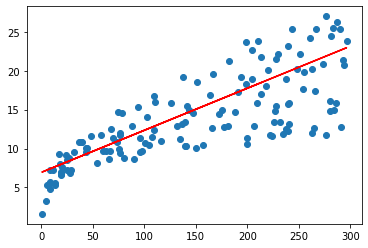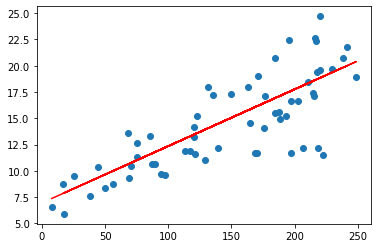# Sales Prediction using Linear Regression in Python

## Introduction

In this blog, we have discussed how to implement the linear regression algorithm to predict sales. From the previous blog, we know that “linear regression” finds the linear relationship between the dependent and independent variables by determining the best fit linear line between them. From the statsmodel library and sklearn library we use the inbuilt linear regression function for prediction.

1. Numpy
2. Pandas
3. Matplotlib
4. Seaborn
5. sklearn
6. statsmodels

## Implementation of Sales Prediction using Linear Regression

### Step-1: Importing Libraries and dataset

# Import the numpy and pandas package

import numpy as np
import pandas as pd

# Data Visualisation

import matplotlib.pyplot as plt
import seaborn as sns

# displays the first 5 rows of the dataset

advertising.head()

### Step-2: Preprocessing the data

In this step, we check for any missing values and outliers and deal with them.

# Checking Null values

# Outlier Analysis

fig, axs = plt.subplots(3, figsize = (5,5))
plt1 = sns.boxplot(advertising['TV'], ax = axs)
plt2 = sns.boxplot(advertising['Newspaper'], ax = axs)
plt.tight_layout()

### Step-3: Exploratory Data Analysis

In this step, we explore the data. Exploratory data analysis (EDA) is a method of data analysis that allows for a precise understanding of the data. Basically, it means being aware of the contents of the data we’re working with.

# with this inbuilt function we can get mean, median, mode etc for the given dataset.

# check for any outliers in target variable

plt.show()

### Step-4: Splitting the dataset

The feature variable TV is first assigned to variable X, and the response variable Sales is assigned to variable Y and split our variable into training and testing sets.

# assigning the variables

# we split our variable into training and testing sets. It is usually a good practice to keep 70% of the data in your train dataset and the rest 30% in your test dataset

from sklearn.model_selection import train_test_split
X_train, X_test, y_train, y_test = train_test_split(X, y, train_size = 0.7, test_size = 0.3, random_state = 100)

### Step-5: Building the model

#### Using statsmodel.api library

First import statsmodel library.  But in order to have an intercept, you need to manually use the add constant attribute of statsmodel. And once you’ve added the constant to your X_Train dataset, you can go ahead and fit a regression line using the OLS.

# importing the library

import statsmodels.api as sm

# Add a constant to get an intercept

# Fit the resgression line using 'OLS'
lr = sm.OLS(y_train, X_train_sm).fit()

# Performing a summary operation lists out all the different parameters of the regression line fitted
print(lr.summary())


#### Using sklearn Library

We use the sklearn library to perform Linear regression. First, we import the Linear Regression module from sklearn and fit the data.

from sklearn import linear_model

# create linear regression object
reg = linear_model.LinearRegression()

X_train= X_train.values.reshape(-1, 1)
y_train= y_train.values.reshape(-1, 1)

# train the model using the training sets
reg.fit(X_train, y_train)

Let’s visualize how well the model fits the data. From the parameters that we get, our linear regression equation becomes Sales=6.948+0.054×TV.

plt.scatter(X_train, y_train)
plt.plot(X_train, 6.948 + 0.054*X_train, 'r')
plt.show()### Step6: Predictions on the Test Set

Making predictions on the test data is the next step after fitting a regression line to your train dataset. To do this, you must first add a constant to the X test data, just as you did for the X train data, and then you can proceed to predict the y values corresponding to the X test using the predicted attribute of the fitted regression line.

# Add a constant to X_test

# Predict the y values corresponding to X_test_sm
y_pred = lr.predict(X_test_sm)

Let us visualize the fit on the test set### Step7: Evaluation Metrics

Evaluation metrics show how well your model is performing.  We use MAE, MSE, RMSE, and R squared error as our evaluation metrics.

from sklearn.metrics import mean_absolute_error,mean_squared_error

mae = mean_absolute_error(y_true=y_test,y_pred=y_pred)
mse = mean_squared_error(y_true=y_test,y_pred=y_pred) #default=True
rmse = mean_squared_error(y_true=y_test,y_pred=y_pred,squared=False)
r_squared = r2_score(y_test, y_pred)

print("MAE:",mae)
print("MSE:",mse)
print("RMSE:",rmse)
print("r_squared",r_squared)